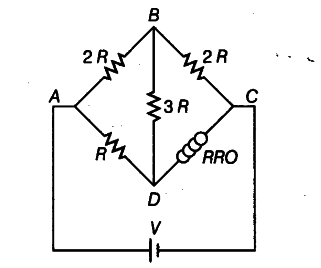# Calculate the value of R in the balance condition of the Wheatstone bridge

Calculate the value of R in the balance condition of the Wheatstone bridge, if the carbon resistor connected across the arm CD has the colour sequence red, red and orange as shown in the figure

(ii) If now the resistance of the arms BC and CD are interchanged, to obtain the balance condition another carbon resistor is connected in place of R. What would now be sequence of colour bands of the carbon resistor? What is the current through the circuit?Let a carbon resistor S is given to the bridge. Then, 2R/R = 2R/S ====> R/S =1
R=S=22 x \${{10}^{3}}\$ Ω = 22 kΩ
(ii)nAfter interchanging the resistance, the balanced bridge would be

2R/X = 22 x \${{10}^{3}}\$/2x22x \${{10}^{3}}\$=1/2
===> X = 4R = 4x22x \${{10}^{3}}\$
=88x \${{10}^{3}}\$Ω
The colour sequence of X is grey,grey and orange.
Thus, equivalent resistance of Wheatstone bridge,

1/\$R_{ eq }\$ = 1/3R + 1/6R = 3/6R
\$R_{ eq }\$ =2R
Current through the circuit, I = 1/3 x v/2R = V/6R A